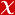## A modified gravity model coupled to a Dirac field in 2D space-times with quadratic nonmetricity and curvature

INTERNATIONAL JOURNAL OF GEOMETRIC METHODS IN MODERN PHYSICS, vol.19, no.03, 2022 (Journal Indexed in SCI)• Publication Type: Article / Article
• Volume: 19 Issue: 03
• Publication Date: 2022
• Doi Number: 10.1142/s0219887822500451
• Title of Journal : INTERNATIONAL JOURNAL OF GEOMETRIC METHODS IN MODERN PHYSICS
• Keywords: Non-Riemannian geometry, gauge theory, Dirac equation, calculation of variation, AFFINE GAUGE-THEORY, DILATON GRAVITY, NON-METRICITY

#### Abstract

After summarizing the basic concepts for the exterior algebra, we first discuss the gauge structure of the bundle over base manifold for deciding the form of the gravitational sector of the total Lagrangian in any dimensions. Then we couple minimally a Dirac spinor field to our gravitational Lagrangian 2-form which is quadratic in the nonmetricity and both linear and quadratic in the curvature in two dimensions. Subsequently, we obtain field equations by varying the total Lagrangian with respect to the independent variables. Finally, we find some classes of solutions of the vacuum theory and then a solution of the Dirac equation in a specific background and analyze them.